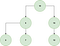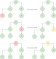# A Developer Revision of Trees

General Trees, Binary Trees, AVL Trees, Red-Black Trees…

This article is aimed at developers with a little prior experience of trees, but who are looking to brush up. It assumes some existing knowledge of Java, as that is the implementation language we will be using, but even those with only a fleeting familiarity should be able to follow along!

This may be a bit of a longer article, as we will dive into implementations of some of the tree types, however you can skip those bits if you just want to get the gist.

I find trees are best described through a diagram.

Each of the green circles represents a node, with the root being the topmost node in the three. This may then have edges connecting it to other nodes. If one node points to another, then the latter node is a child of that parent, where one node removed again is the grandchild (with a similar grandparent relationship).

Nodes all connected to the same parent are known as siblings, and those nodes with no children are leaves. The depth of a node is the vertical distance from the root to the node, whereas the height of the tree is the maximum depth.

Trees have a hierarchical structure as opposed to a linear structure, and if we look at how they are composed we notice that if they have N nodes, they will always have N-1 edges.

The above is an example of a general tree, as there are no constraints on the hierarchy of the tree.

Let’s move into some more specific tree types.A tree that is both a binary and binary search tree

The above is an example of both a binary tree and a binary search tree.

• A binary tree is a tree with at most two children, which are referred to as their left and right nodes.
• A binary search tree keeps values in sorted order. The left node has a value smaller than the parent node which has a value smaller than the right node.

Another useful idea is that of balanced trees. In the binary case this is defined as when the difference in heights of the left and right subtrees for any node is not more than one.

Our previous example was a balanced tree, but let’s have a look at a binary tree that isn’t balanced.

The above is unbalanced, as we can see there is one node where the difference in height between the left and right nodes is greater than one!

We use balanced trees to optimise search times. An unbalanced tree could potentially just be a list, with search time `O(n)` , whereas a balanced tree will be `O(log(n))`.

Let’s take these concepts and use them to introduce a few more, namely insertion, traversals, searches, deletion, checking balance and balancing. The last section will lead us nicely onto self-balancing trees.

The reason that we start with insertion is it allows us to create the tree we described previously, that we can then later use to demonstrate other concepts. We won’t be too worried about balancing for the time being, we can circle back to it later.

We will start with searching in a binary (not search) tree, then move onto search trees. There are a couple of ways we can search a tree:

1. Depth First Search (DFS): In this technique we go as far down each branch as we can before going back up.
2. Breadth First Search (BFS): In this technique we explore the tree level by level.

When to use each method depends on the problem. If we know a solution is close to the root, or the tree is very deep we will want to use BFS. If a tree is very wide or we need to be conscious of memory we may want to use DFS. As we will see in our later implementation BFS uses a queue, and so will be more memory intensive.

Another concept to be wary of for DFS is pre-order vs. in-order vs. post-order traversal. This alludes to the sequence in which we visit the nodes in a tree.

• Pre-order we visit the current node first, before we visit the left and right nodes.
• In-order we visit the left node, the current node, then the right node.
• Post-order we visit the left node, the right node, then the current node.

We have augmented our node to implement each of these below:

BFS is slightly more involved and includes a queue.

Because of the constraints put on the formation of a binary search tree it is relatively easy to find values in its structure. If the current node value is less than the value we are searching for we turn right, if it is equal we have found it, if it is greater we turn left.

Deletion has three cases to deal with:

1. When there are no children we remove the node.
2. When there is one child we move that child up to replace the node.
3. When there are two children we look for the smallest node in the right hand tree and use that to replace the node we intend to delete.The examples shows us deleting a node with no children, then with one child, then with two children

We previously explored the definition of a balanced tree.

This is defined as when the difference in heights of the left and right subtrees for any node is not more than one.

To check this we go down each node and check if the node itself is balanced.

Balancing binary trees is often addressed through AVL trees. AVL Trees keep a record of the difference between the left and right subtrees in a Node (in the format right subtract left), then use this to keep the tree in balance. We’ve added the balanced numbers to our tree diagram below.

This tree is balanced, as none of the nodes have a difference of greater than one.

There are four cases we need to worry about, inserting at a left node on the left side, at a right node on the left side, at a left node on the right side, and at a right node at the right side.

Inserting at a left node on the right side is one of the simplest. Pretend we have done a normal binary tree insertion, leading to the below imbalance. We rotate this away in the manner described below.

Insertion at a right node is slightly trickier. We require an extra rotation to get our nodes into a similar shape to previously, demonstrated below.